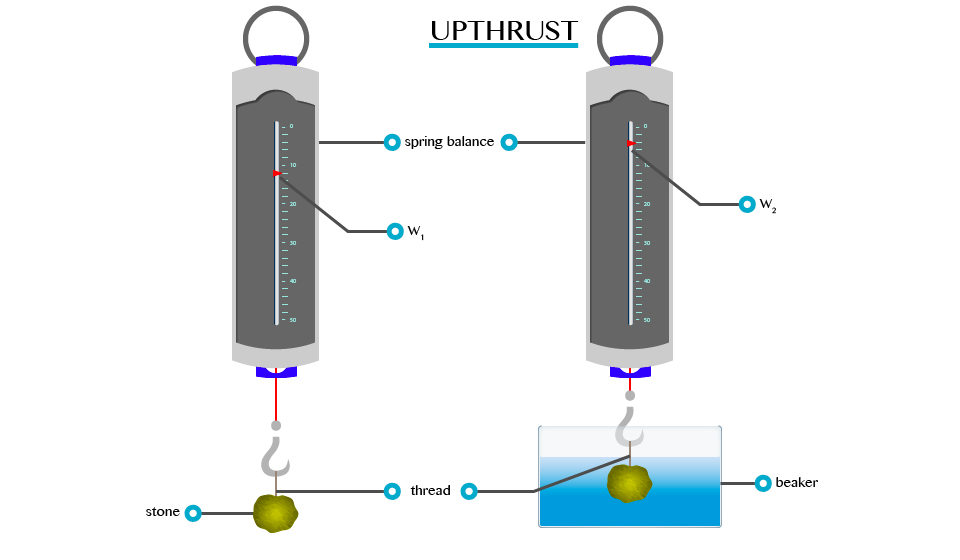# PHYSICS FORM ONE TOPIC 5: ARCHIMEDES’ PRINCIPLE AND LAW OF FLOTATION

Posted on

PHYSICS FORM ONE TOPIC 5: ARCHIMEDES’ PRINCIPLE AND LAW OF FLOTATION

## WARNING Beware of Job Scammers! Please Never Pay Money To Get A Job!

ARCHIMEDES’ PRINCIPLE AND LAW OF FLOTATION

Archimedes’ principle indicates that the upward buoyant force that is exerted on a body immersed in a fluid, whether fully or partially submerged, is equal to the weight of the fluid that the body displaces.
The Concept of Upthrust
Explain the concept of upthrust
If a heavy object is lifted while immersed in water, it may be raised more easily than when outside the water. This is due to the reason that when anything is placed in liquid, it receives an upward force called upthrust. A body appears to have lost some weight when immersed in water due to the upthrust exerted on the body by the water.
• By definition upthrust is referred as an upward force exerted by the body when it’s partially or totally immersed in water.
• Consider the experiment below to verity the concept of upthrust.
From this experiment, it will be observed that W1>W2. This is because:When a body is partially or totally immersed in any liquid, the liquid exerts an upward force.
A weight recorded on the spring balance of a body that is totally or partially immersed in any liquid is called apparent weight. E.g. W2 and the force, which temporally reduces the weight of the body, are called upthrust (u).
Verification of the Archimedes’ Principle
Verify the archimedes principle
This is the principle which shows the relationship between the upthrust acting on a body and the weight of fluid it displaces when partially or completely immersed in the fluid. It was first discovered by a Greek scientist called Archimedes (287 to 121 BC).
• The principle states that, ”when a body is partially or totally immersed in a fluid it experiences an upthrust which is equal to the weight of the fluid displaced.”
• This principle can be verified by the following experiment.
Results:Weight of a body in air = W1Verification of the Archimedes’ Principle
Weight of a body in water = W2 (apparent weight)
Uptrust = Loss of weight in water
U = W1 –W2
Example 1
Weight of a body in air = 10.0N
Weight of a body when immersed in water = 9.2N find the upthrust.
Solution:
Data given
Weight of a body in air (W1)= 10.0N
Weight of a body when in water (W2) = 9.2N
Formula
Upthrust = Loss of weight in water = W1-W2
= 10.0N – 9.2N
= 0.8N
The upthrust is 0.8
Example 2
The weight of a body when totally immersed in liquid is 4.2N. if the weight of the liquid displaced is 2.5N, find the weight of the body in the air.
Solution;
Data;
Apparent weight (W2) = 4.2N
Weight of liquid displaced (u) = 2.5N
Weight of body in air=?
Formular;
U = W1 –W2
W1= 4.2 +2.5 = 6.7N
Weight of body in air is 6.7N
The Archimedes’ Principle in Determining Relative Density
PHYSICS FORM ONE TOPIC 5: ARCHIMEDES’ PRINCIPLE AND LAW OF FLOTATION
Apply the archimedes principle to determine relative density
Relative density (R.D) of a substance can be defined as a ratio of the mass of a certain volume of the substance to the mass of an equal volume of water.
Relative density = Mass or weight of given volume of a substance overMass or weight of an equal volume of water.
R.D = weight of a substance in air over Weight of displaced water.
R.D = weight of a substance in air
From Archimedes’ principle the weight of an equal volume of water is equal to the weight of water displaced by the object, which is equal to the upthrust loss in weight.
By weighing an object in air and then in water, the relative density can be determined.
Weight of a body in air = W1
Weight of a body in water = W2
Apparent loss in weight = W1-W2
Relative density = W1/W1 – W2
Example 3
A piece of glass weigh 1.2N in air and 0.7N when completely immersed water. Calculate its:
1. Relative density
2. Density of a glass
Given that density of water = 1000kg/cm3
And acceleration due to gravity = 10N/kg
Solution
Weight of the glass in air (W1) = 1.2N
Weight of the glass in water (W2) = 0.7N
R.D = W1/W1 – W2
1.2N/(1.2 – 0.7)
1.2/0.5
R.D = 2.4
R.D = Density of glass/Density of water
Density of a glass = R.D x Density of water
= 2.4 x 1000kg/cm
= 2400kg/m3
NB: Relative density has no SI unit